# Networks

This document gives examples of common networks used in parallel computers. For each example the diameter, valency, d×v and bisection bandwidth values are given. Where possible simple expressions are given to show how these parameters vary with the number of processors (P).

# Direct Networks

Direct networks are networks of processing elements(PEs). Although dedicated routing processors may be present, each is associated with a single PE.

### Open Networks

Open networks will usually have spare (dangling) links at the edges allowing for easy expansion.
• Line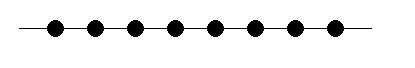P=8 Diameter 7 P-1 Valency 2 2 d×v 14 2(P-1) Bisection Bandwidth B B

• 2D Grid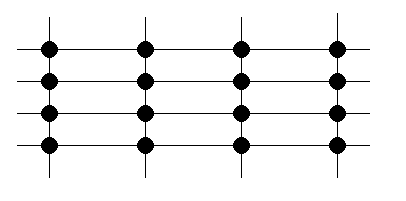P=16 Diameter 6 2(P1/2-1) Valency 4 4 d×v 24 8(P1/2-1) Bisection Bandwidth 4B P1/2B

• 3D Grid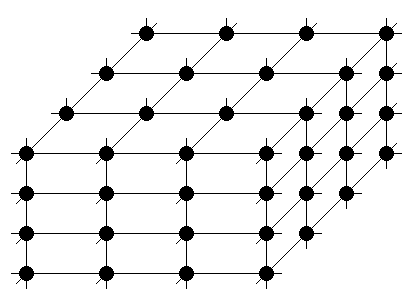Hidden nodes and associated links not shown. P=64 Diameter 9 3(P1/3-1) Valency 6 6 d×v 54 18(P1/3-1) Bisection Bandwidth 16B P2/3B

• ND Grid

Grids of greater than 3 dimensions are unlikely to be encountered.

 Diameter N(P1/N-1) Valency 2N d×v 2N2(P1/N-1) Bisection Bandwidth P(N-1)/NB

• Binary Tree

A binary tree is one in which each non-leaf node has two children.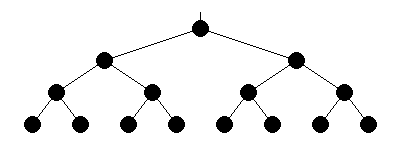P=15 Diameter 6 2log2((P+1)/2) Valency 3 3 d×v 18 6log2((P+1)/2) Bisection Bandwidth B B

Note that ((P+1)/2) is the number of leaf nodes.

• Ternary Tree (N-ary tree with N=3)

In a ternary tree each non-leaf node has three children.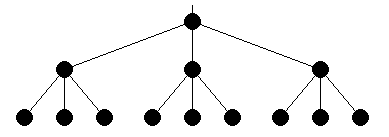P=13 Diameter 4 Valency 4 d×v 16 Bisection Bandwidth 3B

Note that the bisection bandwidth gives the impression that this network performs better than it does. In fact it's just difficult to find somewhere to cut it in half.

• Quaternary Tree (N-ary tree with N=4)

In a quaternary tree each non-leaf node has four children.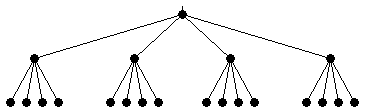P=21 Diameter 4 Valency 5 d×v 20 Bisection Bandwidth 2B

### Closed Networks

Closed Networks have no spare (dangling) links.
• Ring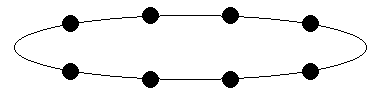P=8 Diameter 4 P/2 Valency 2 2 d×v 8 P Bisection Bandwidth 2B 2B

• 2D Torus

A 2D torus is a 2D grid with the dangling links connected to make ring connections in each dimension. c.f. a ring network is a line with the dangling links connected.

The simple 2D Torus has the shape of the surface of a doughnut.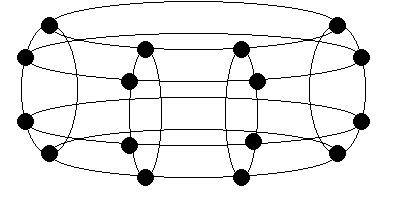P=16 Diameter 4 P1/2 Valency 4 4 d×v 16 4P1/2 Bisection Bandwidth 8B 2P1/2B

• 3D Torus

A 3D torus is a 3D grid with the dangling links connected to make ring connections in each dimension.Hidden nodes and associated links not shown. P=64 Diameter 6 3/2×P1/3 Valency 6 6 d×v 36 9P1/3 Bisection Bandwidth 32B 2P2/3B

• ND Torus

Tori of greater than 3 dimensions are unlikely to be encountered.

 Diameter N/2×P1/N Valency 2N d×v N2P1/N Bisection Bandwidth 2P(N-1)/NB

• ND Binary Hypercube

A binary hypercube is an N dimensional grid in which each side has only two nodes. Note that a cube is considered to be an ND hypercube with N=3.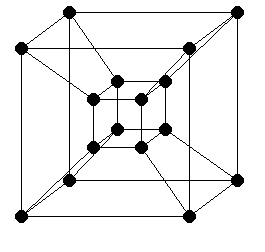N=4,P=16 Diameter 4 log2P=N Valency 4 log2P=N d×v 16 (log2P)2=N2 Bisection Bandwidth 8B PB/2

Bisection bandwidth scales linearly with P

### Uni-directional Networks

• Uni-directional Ring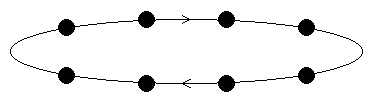P=8 Diameter 7 P-1 Valency 2 2 d×v 14 2(P-1) Bisection Bandwidth B B

# Indirect Networks

Also known as switching networks or dynamic networks. In these networks routing nodes exist independently of processing nodes.

• Binary Fat Tree

A fat tree is one in which the non-root nodes have multiple parents.

In the following binary fat tree each non-root node has two parents.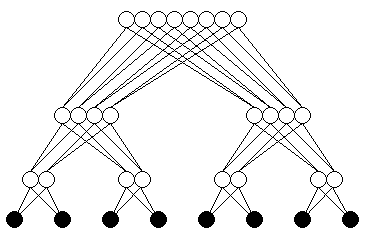P=8 Diameter 6 2log2P Routing Node Valency 4 4 Processing Node Valency 2 2 Bisection Bandwidth 8B PB

Bisection bandwidth scales linearly with P

Alternative representations of the network help to show the increase in bandwidth towards the root of the tree: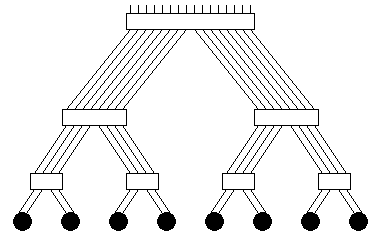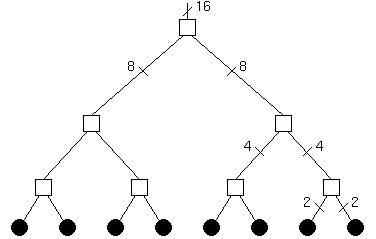• Quaternary Fat Tree (N-ary fat tree with N=4)

In the following quaternary fat tree each non-root node has four parents.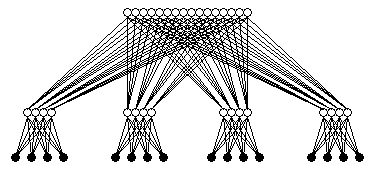P=16 Diameter 4 log2P Routing Node Valency 8 8 Processing Node Valency 4 4 Bisection Bandwidth 32B 2PB

Bisection bandwidth scales linearly with P

• Alternative Quaternary Fat Tree

In the following quaternary fat tree each non-root node has two parents. Note that the 4 in 4-ary (quaternary) refers fo the number of children per parent not the number of parents per child.

Far fewer routing nodes are required in this version.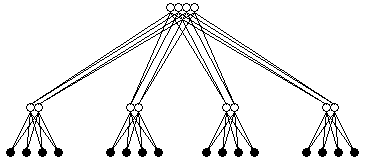P=16 Diameter 4 log2P Routing Node Valency 6 6 Processing Node Valency 2 2 Bisection Bandwidth 8B 2P1/2

Although the diameter is unchanged the bisection bandwidth no longer scales linearly with P.

• N-ary Fat Tree

Where routing processors with more links are available, we can create fat trees with greater N.

In all cases the bisection bandwidth will scale linearly with P provided that each non-root routing node has as many parents as children